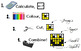# Colouring by Slope & Intercept, 3-CPO (12, 20, and 30 class-size mosaics)Subject
Resource Type
File Type

Zip

(3 MB|133 pages)
Standards
• Product Description
• Standards

Harness the force of collaborative motivation! This is an awesome way for your students to practice finding the slope and y-intercept. The finished product will look great on the wall of your classroom, and students will be proud of their hard work :)

3 mosaic sizes are included, for flexibility of class size:

◾12 worksheet ◾20 worksheet ◾30 worksheet

Calculate... Colour.... Cut... Combine!!

Each student's worksheet is unique (individual accountability!), containing 12 questions in the follow sequence:

First four questions: Find y-intercept from a given slope and point

Next four questions: Find the slope from two given points

Last four questions: Find the y-intercept from two given points

You may wish to require students to find the complete slope-intercept equation for all problems, however.

Check out the product preview to view to know exactly what this task involves!

If you like this product, be sure the check out the whole Collaborative Math Mosaic directory, sorted by topic!

INCLUDED (zip folder)

◾docx and .pdf of everything

◾Full class sets of worksheets (12, 20, and 30) that each combine to create a large collaborative mosaic of 3-CPO

◾Answer keys! See the completed mosaic, the answer range assigned to each colour (i.e. all questions for Blue have an answer between -6 and -2) AND the complete answer key for all questions on every worksheet.

◾Teaching Tips page for smooth implementation

◾Problem Order summary, so you know the sequence of problems on the worksheets.

MATH INVOLVED

◾determine the y-intercept of a line having a given slope and passing through a given point

◾determine the slope of a line passing through two given points

◾determine the y-intercept of a line passing through two given points

Thanks for checking this out! I'd love to hear you feedback, and I love mosaic topic requests!

~CalfordMath

Use similar triangles to explain why the slope m is the same between any two distinct points on a non-vertical line in the coordinate plane; derive the equation 𝘺 = 𝘮𝘹 for a line through the origin and the equation 𝘺 = 𝘮𝘹 + 𝘣 for a line intercepting the vertical axis at 𝘣.
Total Pages
133 pages
Included
Teaching Duration
2 days
Report this Resource to TpT
Reported resources will be reviewed by our team. Report this resource to let us know if this resource violates TpT’s content guidelines.